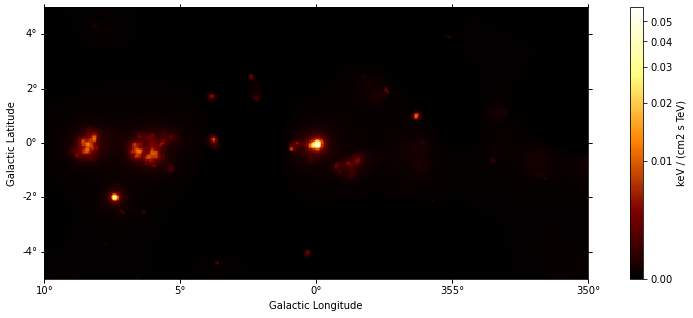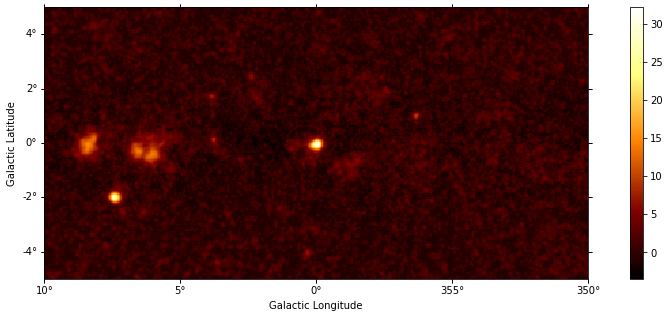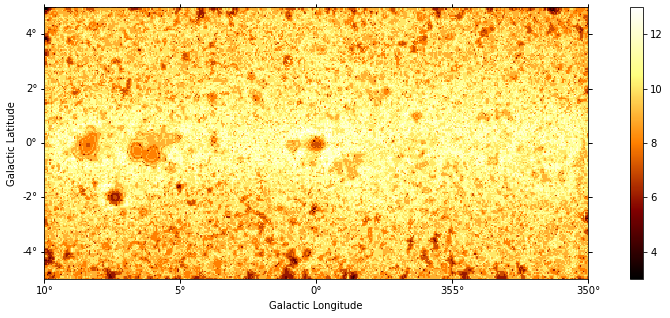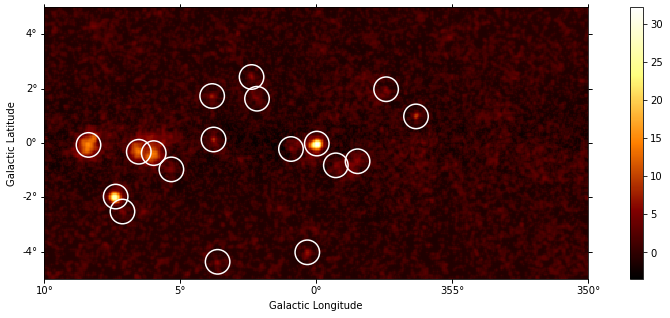This is a fixed-text formatted version of a Jupyter notebook

# Source detection and significance maps¶

## Context¶

The first task in a source catalogue production is to identify significant excesses in the data that can be associated to unknown sources and provide a preliminary parametrization in term of position, extent, and flux. In this notebook we will use Fermi-LAT data to illustrate how to detect candidate sources in counts images with known background.

Objective: build a list of significant excesses in a Fermi-LAT map

## Proposed approach¶

This notebook show how to do source detection with Gammapy using the methods available in gammapy.estimators. We will use images from a Fermi-LAT 3FHL high-energy Galactic center dataset to do this:

• perform adaptive smoothing on counts image

• produce 2-dimensional test-statistics (TS)

• run a peak finder to detect point-source candidates

• compute Li & Ma significance images

• estimate source candidates radius and excess counts

Note that what we do here is a quick-look analysis, the production of real source catalogs use more elaborate procedures.

We will work with the following functions and classes:

• gammapy.maps.WcsNDMap

• gammapy.estimators.ASmoothEstimator

• gammapy.estimators.TSMapEstimator

• gammapy.estimators.utils.find_peaks

## Setup¶

As always, let’s get started with some setup …

:

%matplotlib inline
import matplotlib.pyplot as plt
import matplotlib.cm as cm
from gammapy.maps import Map
from gammapy.estimators import ASmoothMapEstimator, TSMapEstimator
from gammapy.estimators.utils import find_peaks
from gammapy.datasets import MapDataset
from gammapy.modeling.models import (
SkyModel,
PowerLawSpectralModel,
PointSpatialModel,
)
from gammapy.irf import PSFMap, EDispKernelMap
from astropy.coordinates import SkyCoord
import astropy.units as u
import numpy as np


We first read in the counts cube and sum over the energy axis:

:

counts = Map.read(
"$GAMMAPY_DATA/fermi-3fhl-gc/fermi-3fhl-gc-counts-cube.fits.gz" ) background = Map.read( "$GAMMAPY_DATA/fermi-3fhl-gc/fermi-3fhl-gc-background-cube.fits.gz"
)

"$GAMMAPY_DATA/fermi-3fhl-gc/fermi-3fhl-gc-exposure-cube.fits.gz" ) psfmap = PSFMap.read( "$GAMMAPY_DATA/fermi-3fhl-gc/fermi-3fhl-gc-psf-cube.fits.gz",
format="gtpsf",
)

edisp = EDispKernelMap.from_diagonal_response(
energy_axis=counts.geom.axes["energy"],
energy_axis_true=exposure.geom.axes["energy_true"],
)

dataset = MapDataset(
counts=counts,
background=background,
exposure=exposure,
psf=psfmap,
name="fermi-3fhl-gc",
edisp=edisp,
)

WARNING: FITSFixedWarning: 'datfix' made the change 'Set DATEREF to '2001-01-01T00:01:04.184' from MJDREF.
Set MJD-OBS to 54682.655283 from DATE-OBS.
Set MJD-END to 57236.967546 from DATE-END'. [astropy.wcs.wcs]


For visualisation purpose it can be nice to look at a smoothed counts image. This can be performed using the adaptive smoothing algorithm from Ebeling et al. (2006).

In the following example the threshold argument gives the minimum significance expected, values below are clipped.

:

%%time
scales = u.Quantity(np.arange(0.05, 1, 0.05), unit="deg")
smooth = ASmoothMapEstimator(
threshold=3, scales=scales, energy_edges=[10, 500] * u.GeV
)
images = smooth.run(dataset)

CPU times: user 479 ms, sys: 122 ms, total: 602 ms
Wall time: 601 ms

:

plt.figure(figsize=(15, 5))## TS map estimation¶

The Test Statistic, TS = 2 ∆ log L (Mattox et al. 1996), compares the likelihood function L optimized with and without a given source. The TS map is computed by fitting by a single amplitude parameter on each pixel as described in Appendix A of Stewart (2009). The fit is simplified by finding roots of the derivative of the fit statistics (default settings use Brent’s method).

We first need to define the model that will be used to test for the existence of a source. Here, we use a point source.

:

spatial_model = PointSpatialModel()

# We choose units consistent with the map units here...
spectral_model = PowerLawSpectralModel(
amplitude="1e-22 cm-2 s-1 keV-1", index=2
)
model = SkyModel(spatial_model=spatial_model, spectral_model=spectral_model)

:

%%time
estimator = TSMapEstimator(
model,
kernel_width="1 deg",
energy_edges=[10, 500] * u.GeV,
)
maps = estimator.run(dataset)

CPU times: user 5.8 s, sys: 111 ms, total: 5.91 s
Wall time: 5.66 s


### Plot resulting images¶

:

plt.figure(figsize=(15, 5)):

plt.figure(figsize=(15, 5)):

plt.figure(figsize=(15, 5))## Source candidates¶

Let’s run a peak finder on the sqrt_ts image to get a list of point-sources candidates (positions and peak sqrt_ts values). The find_peaks function performs a local maximum search in a sliding window, the argument min_distance is the minimum pixel distance between peaks (smallest possible value and default is 1 pixel).

:

sources = find_peaks(maps["sqrt_ts"], threshold=5, min_distance="0.25 deg")
nsou = len(sources)
sources

:

Table length=18
degdeg
float64int64int64float64float64
32.20620099266.41449-28.97054
27.8365260272.43197-23.54282
15.1713298271.16056-21.74479
14.1436993270.40919-23.47797
13.8828092270.15899-23.98049
9.7642273119263.18257-31.52587
8.7947124102268.46711-25.63326
7.3501123134266.97596-24.77174
6.808619319270.59696-30.69138
6.2444152148265.48068-25.64323
5.876723086266.15140-30.58926
5.665912712272.77351-27.97934
5.6556251139262.90685-30.05853
5.473218195267.17020-28.26173
5.423621483266.78188-29.98429
5.17555749272.82739-24.02653
5.0674156132266.12148-26.23306
5.04479380270.37773-24.84233
:

# Plot sources on top of significance sky image
plt.figure(figsize=(15, 5))

ax.scatter(
sources["ra"],
sources["dec"],
transform=plt.gca().get_transform("icrs"),
color="none",
edgecolor="w",
marker="o",
s=600,
lw=1.5,
);Note that we used the instrument point-spread-function (PSF) as kernel, so the hypothesis we test is the presence of a point source. In order to test for extended sources we would have to use as kernel an extended template convolved by the PSF. Alternatively, we can compute the significance of an extended excess using the Li & Ma formalism, which is faster as no fitting is involve.

## What next?¶

In this notebook, we have seen how to work with images and compute TS and significance images from counts data, if a background estimate is already available.

Here’s some suggestions what to do next:

• Look how background estimation is performed for IACTs with and without the high level interface in analysis_1 and analysis_2 notebooks, respectively

• Learn about 2D model fitting in the modeling 2D notebook

• find more about Fermi-LAT data analysis in the fermi_lat notebook

• Use source candidates to build a model and perform a 3D fitting (see analysis_3d, analysis_mwl notebooks for some hints)

[ ]: